# Tally Charts

Tally Charts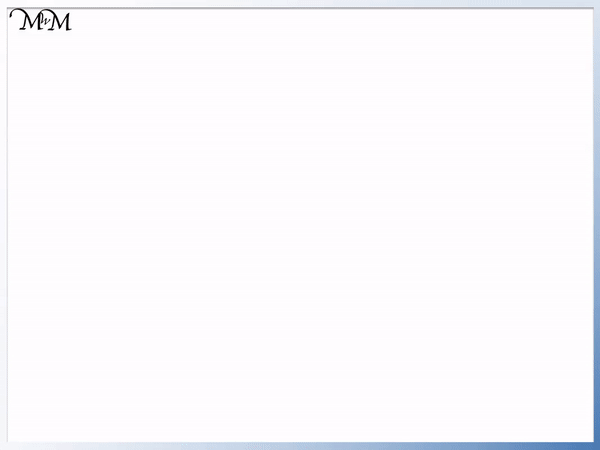• A tally is an easy way to count objects or make a running total.
• A line is drawn as each new number is counted.
• Vertical lines are drawn for the numbers 1 to 4.
• The fifth line is drawn diagonally across the first four to represent the number 5.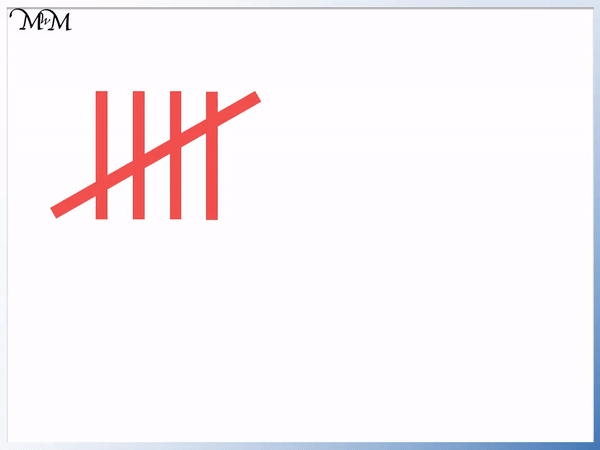• There are four and then a fifth diagonal line is drawn across them.
• Each block of tally marks with a diagonal is a new group of 5.
• Each set of these 5 lines can be counted in fives, moving through the 5 times table.
• Tally marks are easier to count in groups of 5.

A tally is a line drawn as a new number is counted.

Numbers in the five times table are drawn as a diagonal line.• To read this tally we can start by looking at the first block of 5 tally marks.
• The block with a diagonal line across is 5 lines.
• To count the total tally we count on from five.
• There are 3 more marks following the first block of 5.
• We count on from 5: 6, 7 and 8.
• 5 + 3 = 8 and so this tally represents 8.#### Tally Chart Lesson Accompanying Activity Sheet# How to Make and Draw Tally Charts

A tally is used to help count a number of objects and it is particularly useful for making a running total.

A tally mark is a line drawn as each new number is counted.

Below is a tally of the number of counters with a new tally mark drawn as each is counted.To draw a tally, each number from 1 to 4 is drawn with a

, which is a line going from top to bottom.

The number 5 is represented as a tally by drawing a diagonal line across the first four lines.

Making a tally is useful for making a running total. In this example above we have three counters before a fourth counter is added. Updating the tally is easy because another line is simply drawn alongside the first three.

This tally method is easier than writing the number ‘3’ and then crossing it out or erasing it before writing the number ‘4’.

We can continue to count on using a tally past the number 5 as more counters are added.

5 is represented with a diagonal line across the first 4 lines. We only draw one diagonal line across each set of 4.

Once another counter is added, we have 6 counters and so we simply draw another vertical line alongside our group of 5.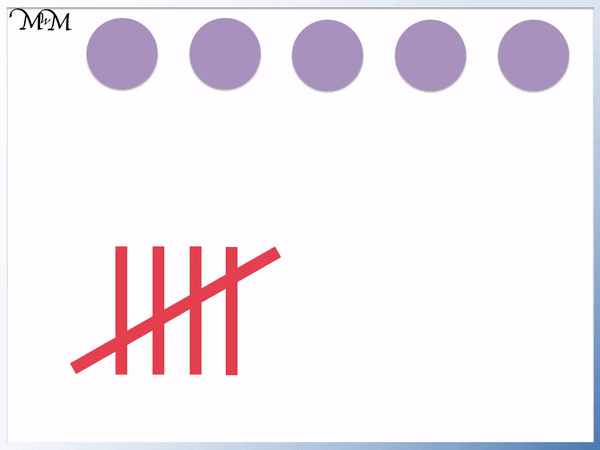We can continue to draw a vertical tally mark for each new number from 6 to 9.

The number 9 is represented as a tally with one group of 5 and four more vertical lines.

We can see that we have 4 free vertical lines.

So the next number will be represented as a tally with a diagonal line across.

Whenever there are 4 vertical lines as a tally, the next tally mark is a diagonal line across them.

The number 10 is in the five times table.

Every number in the five times table is represented with a diagonal line.

This method is used because it allows us to easily count larger numbers as a tally by counting up in groups of 5.

Below are the

shown in tally form. We do not count each individual tally mark, we simply count up in fives.Each group that has a diagonal across it is a new number in the five times table.

These are:

5, 10, 15 and 20.

This method allows us to quickly count a tally.

Below is an example of reading a tally.We know that the first group in the tally which contains a diagonal line is a group of 5.

To count this tally we simply count on from five.

We have three more vertical lines that are free following the group of five and so, we will count on three more from 5.

We have 6, 7 and then 8.

5 + 3 = 8.

And so, this tally represents 8.

We do not count each individual tally mark, we simply count on from 5.

Here is another example of reading a tally.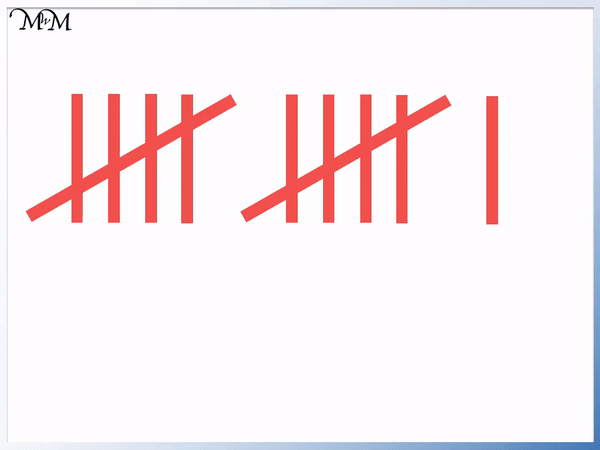We have two groups of 5, which are the two groups of tally marks with diagonals across them.

2 lots of 5 are 10.

And so, we count on from 10.

We have one more free vertical line and therefore we count 1 more than 10, which is 11.

This tally represents 11.

We have seen that tallies are an easy way of representing numbers when counting.

Tally marks are easy to write and are often put into a chart before we count up the total.

A tally chart is a table of tally marks which are then counted. The total number is often called the frequency.

In the tally chart below, 4 people: Dennis, Jenny, Lisa and Ron have scored the following number of points in a game.

Since points in the game are awarded as the game is played, a running total is made. Remember that a tally is a very useful way of counting a running total.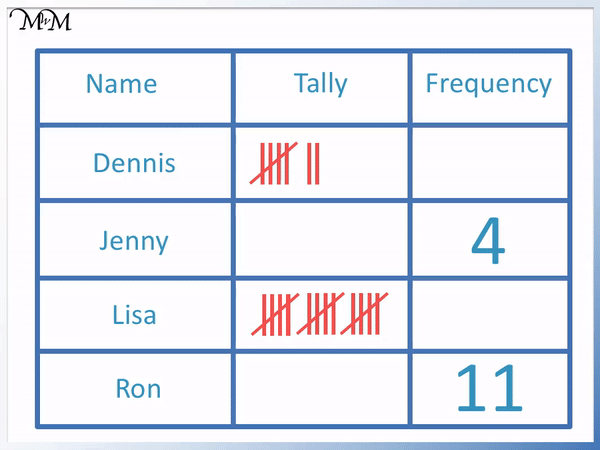Dennis has a group of 5 plus two more.

5 + 2 = 7 and so, Dennis scored 7.

Jenny scored 4, which is represented by four tally marks drawn as vertical lines.

Lisa has exactly three groups of 5.

3 x 5 = 15 and so, Lisa has a score of 15.

Ron scored 11.

Two groups of 5 are 10. Eleven is one more than 10 and so, we draw a single tally mark after the two groups of 5.

Below is another tally chart example which shows the number of people who prefer each colour.The tally for red is shown as three tally marks, which means that 3 people prefer red.

The tally for blue is made of two groups of 5, plus 3 more tally marks.

Two lots of five is 10 and so, we can count on three from 10.

13 people prefer blue.

The tally for green is shown with one more tally mark following the group of 5.

One more than 5 is 6.

6 people prefer green.

The most popular colour is the colour that most people prefer.

We look for the biggest number in the frequency column.

13 is the biggest number and so, blue is the most popular colour.

In this final example we have a tally chart showing the number of goals scored by the following four people.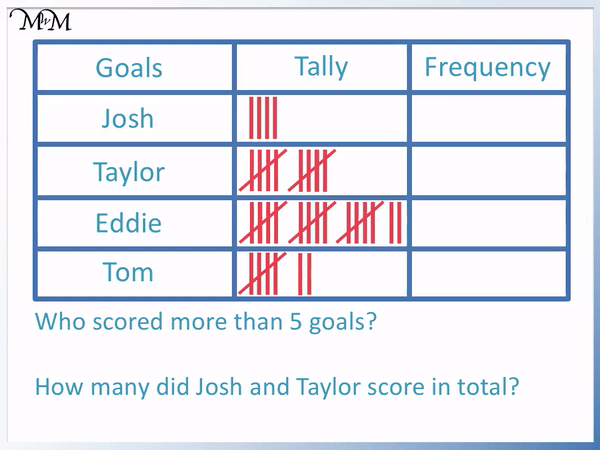Josh has scored 4 goals as shown by 4 vertical tally marks.

Taylor has exactly two groups of 5. Two lots of five are ten.

Taylor has scored 10 goals.

Eddie has three lots of five and then two more.

Three lots of five are fifteen. Two more than 15 is 17.

Eddie has scored 17 goals.

Tom has scored two more than five.

Tom has scored 7 goals.

We are asked, “Who scored more than five goals?”.

The players who has more than five goals are Taylor, Eddie and Tom.

We can see that Josh is the only player who does not have a group of five. We can see that his tally does not have a diagonal line which would represent 5 goals.

We are asked, “How many did Josh and Taylor score in total?”.

We add the numbers shown for Josh and Taylor.

4 + 10 = 14 goals.

We can see that tally charts are a useful way to keep a record of points or scores. They may also be used for counting a running total.

You can make your own tally chart to show the number of cars that go past your house or the number of days that it rains this month.Now try our lesson on Bar Charts where we learn how to draw and interpret bar charts.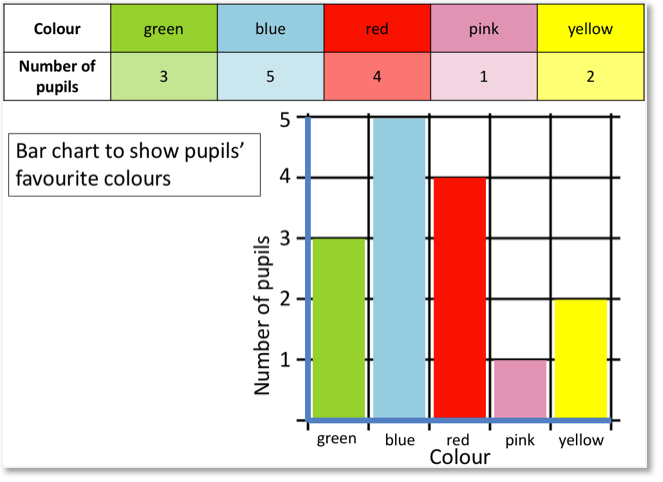error: Content is protected !!